Since R2021a

## Syntax

``scoreMap = gradCAM(net,X,label)``
``scoreMap = gradCAM(net,X,reductionFcn)``
``[scoreMap,featureLayer,reductionLayer] = gradCAM(___)``
``___ = gradCAM(___,Name,Value)``

## Description

example

````scoreMap = gradCAM(net,X,label)` returns the gradient-weighted class activation mapping (Grad-CAM) map of the change in the classification score of input `X`, when the network `net` evaluates the class score for the class given by `label`. Use this function to explain network predictions and check that your network is focusing on the right parts of the data. The Grad-CAM interpretability technique uses the gradients of the classification score with respect to the final convolutional feature map. The parts of an observation with a large value for the Grad-CAM map are those that most impact the network score for that class.Use this syntax to compute the Grad-CAM map for classification tasks.```

example

````scoreMap = gradCAM(net,X,reductionFcn)` returns the Grad-CAM importance map using a reduction function. `reductionFcn` is a function handle that reduces the output activations of the reduction layer to a scalar value. This scalar fulfills the role of the class score for classification tasks, and generalizes the Grad-CAM technique to nonclassification tasks, such as regression. The `gradCAM` function computes the Grad-CAM map by differentiating the reduced output of the reduction layer with respect to the features in the feature layer. `gradCAM` automatically selects reduction and feature layers to use when computing the map. To specify these layers, use the `'ReductionLayer'` and `'FeatureLayer'` name-value arguments. Use this syntax to compute the Grad-CAM map for nonclassification tasks.```
````[scoreMap,featureLayer,reductionLayer] = gradCAM(___)` also returns the names of the feature layer and reduction layer used to compute the Grad-CAM map. Use this syntax with any of the input-argument combinations in previous syntaxes. ```

example

````___ = gradCAM(___,Name,Value)` specifies options using one or more name-value arguments in addition to the input arguments in previous syntaxes. For example, `'ReductionLayer','prob'` sets the reduction layer to the `net` layer named `'prob'`. ```

## Examples

collapse all

Use `gradCAM` to visualize which parts of an image are important to the classification decision of a network.

Import the pretrained network SqueezeNet.

`net = squeezenet;`

Import the image and resize it to match the input size for the network.

```X = imread("laika_grass.jpg"); inputSize = net.Layers(1).InputSize(1:2); X = imresize(X,inputSize);```

Display the image.

`imshow(X)`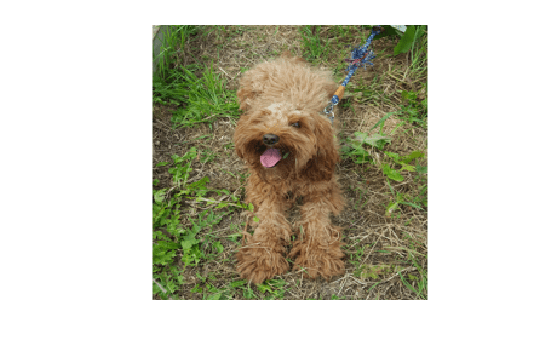Classify the image to get the class label.

`label = classify(net,X)`
```label = categorical toy poodle ```

Use `gradCAM` to determine which parts of the image are important to the classification result.

`scoreMap = gradCAM(net,X,label);`

Plot the result over the original image with transparency to see which areas of the image contribute most to the classification score.

```figure imshow(X) hold on imagesc(scoreMap,'AlphaData',0.5) colormap jet```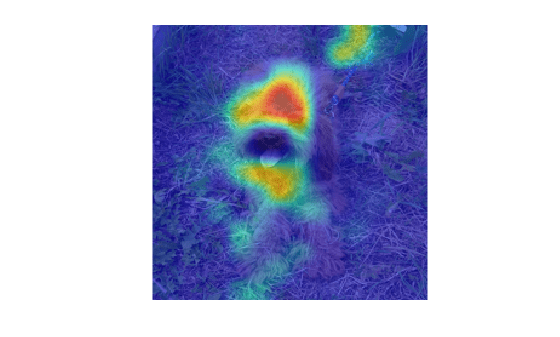The network focuses predominantly on the back of the dog to make the classification decision.

Use Grad-CAM to visualize which parts of an image are most important to the predictions of an image regression network.

Load the pretrained network `digitsRegressionNet`. This network is a regression convolutional neural network that predicts the angle of rotation of handwritten digits.

`load digitsRegressionNet`

View the network layers. The output layer of the network is a regression layer.

`layers = net.Layers`
```layers = 18x1 Layer array with layers: 1 'imageinput' Image Input 28x28x1 images with 'zerocenter' normalization 2 'conv_1' 2-D Convolution 8 3x3x1 convolutions with stride [1 1] and padding 'same' 3 'batchnorm_1' Batch Normalization Batch normalization with 8 channels 4 'relu_1' ReLU ReLU 5 'avgpool2d_1' 2-D Average Pooling 2x2 average pooling with stride [2 2] and padding [0 0 0 0] 6 'conv_2' 2-D Convolution 16 3x3x8 convolutions with stride [1 1] and padding 'same' 7 'batchnorm_2' Batch Normalization Batch normalization with 16 channels 8 'relu_2' ReLU ReLU 9 'avgpool2d_2' 2-D Average Pooling 2x2 average pooling with stride [2 2] and padding [0 0 0 0] 10 'conv_3' 2-D Convolution 32 3x3x16 convolutions with stride [1 1] and padding 'same' 11 'batchnorm_3' Batch Normalization Batch normalization with 32 channels 12 'relu_3' ReLU ReLU 13 'conv_4' 2-D Convolution 32 3x3x32 convolutions with stride [1 1] and padding 'same' 14 'batchnorm_4' Batch Normalization Batch normalization with 32 channels 15 'relu_4' ReLU ReLU 16 'dropout' Dropout 20% dropout 17 'fc' Fully Connected 1 fully connected layer 18 'regressionoutput' Regression Output mean-squared-error with response 'Response' ```

`[XTest,~,YTest] = digitTest4DArrayData;`

Evaluate the performance of the network on a test image.

```testIdx = 501; testDigit = XTest(:,:,:,testIdx);```

Use `predict` to predict the angle of rotation and compare the predicted rotation to the true rotation.

`predRotation = predict(net,testDigit)`
```predRotation = single 26.5635 ```
`trueRotation = YTest(testIdx)`
```trueRotation = 29 ```

Visualize the regions of the image most important to the network prediction using `gradCAM`. Select the ReLU layer as the feature layer and the fully connected layer as the reduction layer.

```featureLayer = 'relu_4'; reductionLayer = 'fc';```

Define the reduction function. The reduction function must reduce the output of the reduction layer to a scalar value. The Grad-CAM map displays the importance of different parts of the image to that scalar. In this regression problem, the network predicts the angle of rotation of the image. Therefore, the output of the fully connected layer is already a scalar value and so the reduction function is just the identity function.

`reductionFcn = @(x)x;`

```scoreMap = gradCAM(net,testDigit,reductionFcn, ... 'ReductionLayer',reductionLayer, ... 'FeatureLayer',featureLayer);```

Display the Grad-CAM map over the test image.

```ax(1) = subplot(1,2,1); imshow(testDigit) title("True Rotation = " + trueRotation + '\newline Pred Rotation = ' + round(predRotation,0)) colormap(ax(1),'gray') ax(2) = subplot(1,2,2); imshow(testDigit) hold on imagesc(rescale(scoreMap)) colormap(ax(2),'jet') title("Grad-CAM") hold off```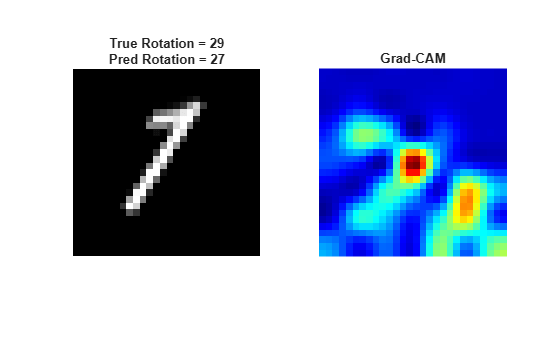Use `gradCAM` to visualize which parts of a sequence are important to the classification decision of a network.

Load the pretrained network `JapaneseVowelsConvNet`. This network is a pretrained 1-D convolutional neural network trained on the Japanese Vowels data set as described in  and .

`load JapaneseVowelsConvNet`

View the network architecture.

`net.Layers`
```ans = 11x1 Layer array with layers: 1 'sequenceinput' Sequence Input Sequence input with 12 dimensions 2 'conv1d_1' 1-D Convolution 32 3x12 convolutions with stride 1 and padding 'causal' 3 'relu_1' ReLU ReLU 4 'layernorm_1' Layer Normalization Layer normalization with 32 channels 5 'conv1d_2' 1-D Convolution 64 3x32 convolutions with stride 1 and padding 'causal' 6 'relu_2' ReLU ReLU 7 'layernorm_2' Layer Normalization Layer normalization with 64 channels 8 'globalavgpool1d' 1-D Global Average Pooling 1-D global average pooling 9 'fc' Fully Connected 9 fully connected layer 10 'softmax' Softmax softmax 11 'classoutput' Classification Output crossentropyex with '1' and 8 other classes ```

Load the test data. `XTest` is a cell array containing sequences of dimension 12 of varying length. `TTest` is a categorical vector of labels "1","2",...,"9", which correspond to the nine speakers.

`[XTest,TTest] = japaneseVowelsTestData;`

Select the first time series observation. This sequence has 19 time steps.

```testSequence = XTest{1}; testSize = size(testSequence)```
```testSize = 1×2 12 19 ```
`numFeatures = testSize(1);`

Visualize the first time series in a plot. Each line corresponds to a feature.

```figure plot(testSequence') title("Test Observation 1") xlabel("Time Step") legend("Feature " + string(1:numFeatures),'Location','northeastoutside')```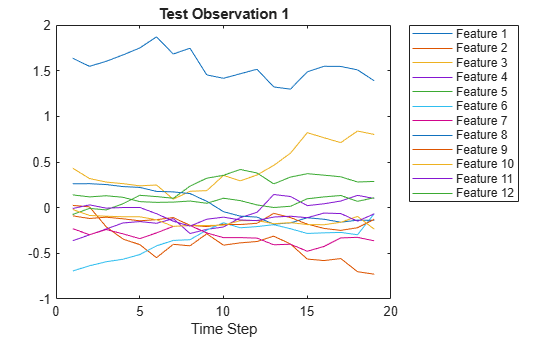Classify the test observation.

`label = classify(net,testSequence)`
```label = categorical 1 ```

Use `gradCAM` to determine which parts of the sequence are important to the classification result.

`map = gradCAM(net,testSequence,label);`

`map` is a 1-by-19 vector containing the importance value for each of the 19 time steps.

Plot the results to see which time steps contribute most to the classification score.

```figure subplot(2,1,1) plot(testSequence') xlabel("Time Step") subplot(2,1,2) plot(map) xlabel("Time Step") ylabel("Grad-CAM Importance")```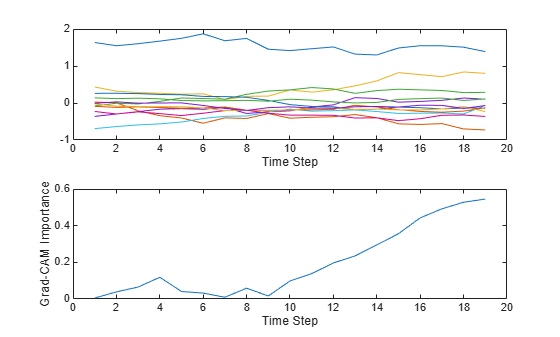The Grad-CAM map suggests that the network is focusing on the later time steps to make the classification decision.

## Input Arguments

collapse all

Trained network, specified as a `SeriesNetwork`, `DAGNetwork`, or `dlnetwork` object. You can get a trained network by importing a pretrained network or by training your own network using the `trainNetwork` function or custom training. For more information about pretrained networks, see Pretrained Deep Neural Networks.

`net` must contain a single input layer and a single output layer. The input layer of `net` must be an `imageInputLayer`, `image3dInputLayer`, or `sequenceInputLayer`. For networks with a `sequenceInputLayer`, the `InputSize` property must be scalar.

Input data, specified as a numeric array or formatted `dlarray` object.

• For 2-D image data, `X` must be a h-by-w-by-c array, where h, w, and c are the height, width, and number of channels of the network input layer, respectively.

• For 3-D image data, `X` must be a h-by-w-by-d-by-c array, where h, w, d, and c are the height, width, depth, and number of the network input layer, respectively.

• For vector sequence data, `X` must be a c-by-t array, where `c` is the input size of the network `net` and `t` is the number of time steps in the sequence and can be any positive integer.

Data Types: `single` | `double` | `int8` | `int16` | `int32` | `int64` | `uint8` | `uint16` | `uint32` | `uint64`

Class label to use for calculating the Grad-CAM map for classification and semantic segmentation tasks, specified as a categorical, a character vector, a string scalar, a numeric index, or a vector of these values.

For `dlnetwork` objects, you must specify `label` as a categorical or a numeric index.

If you specify `label` as a vector, the software calculates the feature importance for each class label independently. In that case, `scoreMap` contains the map for each class in `label`. For more information, see `scoreMap`.

The `gradCAM` function sums over the spatial and time dimensions of the reduction layer for class `label`. Therefore, you can specify `label` as the classes of interest for semantic segmentation tasks and `gradCAM` returns the Grad-CAM importance for each pixel.

Example: `["cat" "dog"]`

Example: `[1 5]`

Data Types: `char` | `string` | `categorical`

Reduction function, specified as a function handle. The reduction function reduces the output activations of the reduction layer to a single value and must reduce a `dlarray` object to a `dlarray` scalar. This scalar fulfills the role of `label` in classification tasks, and generalizes the Grad-CAM technique to nonclassification tasks, such as regression.

Grad-CAM uses the reduced output activations of the reduction layer to compute the gradients for the importance map.

Example: `@x(x)`

Data Types: `function_handle`

### Name-Value Arguments

Specify optional pairs of arguments as `Name1=Value1,...,NameN=ValueN`, where `Name` is the argument name and `Value` is the corresponding value. Name-value arguments must appear after other arguments, but the order of the pairs does not matter.

Before R2021a, use commas to separate each name and value, and enclose `Name` in quotes.

Example: `'FeatureLayer','conv10','ReductionLayer','prob','OutputUpsampling','bicubic','ExecutionEnvironment','gpu'` computes the Grad-CAM map with respect to layers `'conv10'` and `'prob'`, executes the calculation on the GPU, and upsamples the resulting map to the same size as the input data using bicubic interpolation.

Name of the feature layer to extract the feature map from when computing the Grad-CAM map, specified as a string or character vector. For most tasks, use the last ReLU layer with nonsingleton spatial or time dimensions, or the last layer that gathers the outputs of ReLU layers (such as depth concatenation or addition layers). If your network does not contain any ReLU layers, specify the name of the final convolutional layer that has nonsingleton spatial or time dimensions in the output.

The default value is the final layer with nonsingleton spatial or time dimensions. Use the `analyzeNetwork` function to examine your network and select the correct layer.

Example: `'FeatureLayer','conv10'`

Data Types: `char` | `string`

Name of the reduction layer to extract output activations from when computing the Grad-CAM map, specified as a string or character vector. For classification tasks, this layer is usually the final softmax layer. For other tasks, this layer is usually the penultimate layer for DAG and series networks and the final layer for `dlnetwork` objects.

The default value is the penultimate layer in DAG and series networks, and the final layer in `dlnetwork` objects. Use the `analyzeNetwork` function to examine your network and select the correct layer.

Example: `'ReductionLayer','prob'`

Data Types: `char` | `string`

Data format assigning a label to each dimension of the input data, specified as a character vector or a string. Each character in the format must be one of the following dimension labels:

• `S` — Spatial

• `C` — Channel

• `B` — Batch

• `T` — Time

For more information, see `dlarray`.

The `gradCAM` function supports at most one singleton batch dimension.

Example: `'Format','SSC'`

Data Types: `char` | `string`

Output upsampling method, specified as the comma-separated pair consisting of` 'OutputUpsampling'` and one of the following values:

• `'bicubic'` — Use bicubic interpolation to produce a smooth map.

• `'nearest'` — Use nearest-neighbor interpolation to expand the map.

• `'none'` — Use no upsampling. The map can be smaller or larger than the input data.

If `'OutputUpsampling'` is `'nearest'` or `'bicubic'`, the Grad-CAM map is the same size as the spatial and temporal dimensions of the input.

• For 2-D image data, the map is size h-by-w, where h and w are the height and width of the image, respectively

• For 3-D image data, the map is size h-by-w-by-d, where h, w, and d are the height, width, and depth of the image, respectively.

• For vector sequence input, the map is size 1-by-t, where t is the number of time steps in the sequence.

If `'OutputUpsampling'` is `'nearest'` or `'bicubic'`, the computed map is upsampled using the `imresize` function for 2-D image and vector sequence data, and the `imresize3` (Image Processing Toolbox) function for 3-D image data. For 3-D image data, the option `'bicubic'` uses `imresize3` (Image Processing Toolbox) with the `'cubic'` method.

Example: `'OutputUpsampling','bicubic'`

Hardware resource for computing the map, specified as the comma-separated pair consisting of `'ExecutionEnvironment'` and one of the following.

• `'auto'` — Use the GPU if one is available. Otherwise, use the CPU.

• `'cpu'` — Use the CPU.

• `'gpu'` — Use the GPU.

The GPU option requires Parallel Computing Toolbox™. To use a GPU for deep learning, you must also have a supported GPU device. For information on supported devices, see GPU Computing Requirements (Parallel Computing Toolbox). If you choose the `'gpu'` option and Parallel Computing Toolbox and a suitable GPU are not available, then the software returns an error.

Example: `'ExecutionEnvironment','gpu'`

## Output Arguments

collapse all

Grad-CAM importance map, returned as a row vector, numeric matrix or a numeric array. Areas in the map with higher positive values correspond to regions of input data that contribute positively to the prediction.

• For classification tasks, `scoreMap` is the gradient of the final classification score for the specified class, with respect to each feature in the feature layer.

• For other types of tasks, `scoreMap` is the gradient of the reduced output of the reduction layer, with respect to each feature in the feature layer.

The size of `scoreMap` depends on the type of data.

• For 2-D image data, `scoreMap` is a matrix. `scoreMap(i,j)` corresponds to the Grad-CAM importance at the spatial location `(i,j)`. If you provide `label` as a vector of categoricals, character vectors, or strings, then `scoreMap(:,:,n)` corresponds to the map for `label(n)`.

• For 3-D image data, `scoreMap` is a 3-D array. `scoreMap(i,j,k)` corresponds to the Grad-CAM importance at the spatial location `(i,j,k)`. If you provide `label` as a vector of categoricals, character vectors, or strings, then `scoreMap(:,:,:,n)` corresponds to the map for `label(n)`.

• For vector sequence data, `scoreMap` is a row vector. `scoreMap(:,i)` corresponds to the Grad-CAM importance at time step `i`. If you provide `label` as a vector of categoricals, character vectors, or strings, then `scoreMap(:,:,n)` corresponds to the map for `label(n)`.

Name of the feature layer to extract the feature map from when computing the Grad-CAM map, returned as a string.

By default, `gradCAM` chooses a feature layer to use to compute the Grad-CAM map. This layer is the final layer with nonsingleton spatial or time dimensions. You can specify which feature layer to use using the `'FeatureLayer'` name-value argument. When you specify the `'FeatureLayer'` name-value argument, `featureLayer` returns the same value.

Name of the reduction layer to extract output activations from when computing the Grad-CAM map, returned as a string.

By default, `gradCAM` chooses a reduction layer to use to compute the Grad-CAM map. This layer is the penultimate layer in DAG and series networks, and the final layer in `dlnetwork` objects. You can also specify which reduction layer to use using the `'ReductionLayer'` name-value argument. When you specify the `'ReductionLayer'` name-value argument, `reductionLayer` returns the same value.

collapse all

Gradient-weighted class activation mapping (Grad-CAM) is an explainability technique that can be used to help understand the predictions made by a deep neural network . Grad-CAM, a generalization of the CAM technique, determines the importance of each neuron in a network prediction by considering the gradients of the target flowing through the deep network.

Grad-CAM computes the gradient of a differentiable output, for example class score, with respect to the convolutional features in the chosen layer. The gradients are pooled over space and time dimensions to find the neuron importance weights. These weights are then used to linearly combine the activation maps and determine which features are most important to the prediction.

Suppose you have a 2-D image classification network with output yc, representing the score for class c, and want to compute the Grad-CAM map for a convolutional layer with k feature maps (channels), Aki,j, where i,j indexes the pixels. The neuron importance weight is

where N is the total number of pixels in the feature map. The Grad-CAM map is then a weighted combination of the feature maps with an applied ReLU:

`$M=\text{ReLU}\left({\sum }_{k}{\alpha }_{k}^{c}{A}^{k}\right).$`

The ReLU activation ensures you get only the features that have a positive contribution to the class of interest. The output is therefore a heatmap for the specified class, which is the same size as the feature map. The Grad-CAM map is then upsampled to the size of the input data.

Although Grad-CAM is commonly used for classification tasks, you can compute a Grad-CAM map for any differentiable activation. For example, for semantic segmentation tasks, you can calculate the Grad-CAM map by replacing yc with ${\sum }_{\left(i,j\right)\in S}{y}_{ij}^{c}$, where S is the set of pixels of interest and yi,jc is 1 if pixel (i,j) is predicted to be class c, and 0 otherwise . You can use the `gradCAM` function for nonclassification tasks by specifying a suitable reduction function that reduces the output activations of the reduction layer to a single value and takes the place of yc in the neuron importance weight equation.

## Tips

• The `reductionFcn` function receives the output from the reduction layer as a traced `dlarray` object. The function must reduce this output to a scalar `dlarray`, which `gradCAM` then differentiates with respect to the activations of the feature layer. For example, to compute the Grad-CAM map for channel 208 of the softmax activations of a network, the reduction function is `@(x)(x(208))`. This function receives the activations and extracts the 208th channel.

• The `gradCAM` function automatically chooses reduction and feature layers to use when computing the Grad-CAM map. For some networks, the chosen layers might not be suitable. For example, if your network has multiple layers that can be used as the feature layer, then the function chooses one of those layers, but its choice might not be the most suitable. For such networks, specify which feature layer to use using the `'FeatureLayer'` name-value argument.

• To display the Grad-CAM map as an overlay on a grayscale image, you must first rescale the map to be in the range [0, 1]. For example, ```scoreMapRescaled = rescale(scoreMap);```.

 Kudo, Mineichi, Jun Toyama, and Masaru Shimbo. “Multidimensional Curve Classification Using Passing-through Regions.” Pattern Recognition Letters 20, no. 11–13 (November 1999): 1103–11. https://doi.org/10.1016/S0167-8655(99)00077-X.

 UCI Machine Learning Repository: Japanese Vowels Dataset. https://archive.ics.uci.edu/ml/datasets/Japanese+Vowels.

 Selvaraju, Ramprasaath R., Michael Cogswell, Abhishek Das, Ramakrishna Vedantam, Devi Parikh, and Dhruv Batra. “Grad-CAM: Visual Explanations from Deep Networks via Gradient-Based Localization.” 2017 (October 2017): 618–626, https://doi.org/10.1109/ICCV.2017.74.

 Vinogradova, Kira, Alexandr Dibrov, and Gene Myers. “Towards Interpretable Semantic Segmentation via Gradient-Weighted Class Activation Mapping.” Proceedings of the AAAI Conference on Artificial Intelligence 34, no. 10 (April 2020): 13943–13944, https://doi.org/10.1609/aaai.v34i10.7244.